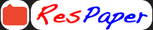Trending ▼   ResFinder# ICSE Prelims 2018 : Biology (Sri Sri Ravishankar Vidya Mandir (SSRVM), Mumbai)

7 pages, 91 questions, 90 questions with responses, 289 total responses,20Science Jeannie +Fave Message ProfileTimelineUploads
 Home > jeannie13 >   F Also featured on: School Page icse and 1 moreFormatting page ...

m tn a r g E S td S u e c t: B io lo g y D a te 0 9 / 0 2 0 1 8 io x t ]u r t tn a 20 17 18 n M X a rk s T im 80 2 H rs e t l th e p a p e r p r o v id e d s e p a r a e y to th is P a p e r m u s t b e w r itte n o n in u te s 5 lo w e d to w r ite d u r in g th e firs t 1 m Y o u w il n o t b e a s tio n p a p e r T h is tim e is to b e s p e n t in re a d in g th e q u e h n s w e rs o w e d fo r w r itin g t e a is th e tim e a T h e tim e g iv e n a t th e h e a d o f th is p a p e r A n s w e rs tio n s fro m S e c tio n q u e s tio n s fr o m S e c tio n I a n d a n y fo u r q u e s A e m pt a tio n s a re g iv e n in b r a c k e ts [ ] T h e in te n d e d m a r k s fo r q u e s tio n s o r p a r ts o f q u e s (A tte m O N (4 0 M a r k s ) S EC T l q u e s ti n s fro m th is S e c tio pt a n ) es " e th e [5 fo llo w in g r T h e p h o to re c e p to t T h e h o rm rs s e n s th a t s tim o ne itiv e to b rig h t lig h t u la te s th e re le a s e o f g lu in to th e b lo co se o d . A n e u ro tra n s m o n e (b ) R e w r ite a n Ea u r ith e d A r co m u ' X ' o r r ' Y r e te r : tr a n s :: ( bi B le e d in g C :: lr c y to k in e s s : ll s a p " t" " " e " " " " " " " ly [5 ] d p a ir u rin e H e a rt: p e ric a rd iu m e " h i" E ye n e rv e ts po t in th e b o d y " " secon Ui se n c h ro m o s o m e ' e te th e p d ito ry p re f" o am nm " " itte d iv is io n : tra n s p o r ts te a rs b ra in G u o f c y to p la s m tta tio n :: K a ry o k in e s is Scanned by CamScannerFormatting page ...

Top Contributors
to this ResPaperPranayak Uniyal(100)Sparsh Agarwal(83)Pranav Ajinkya(77)Shreyas Pattanshetty(49)Formatting page ...Formatting page ...Formatting page ...Formatting page ...Formatting page ...